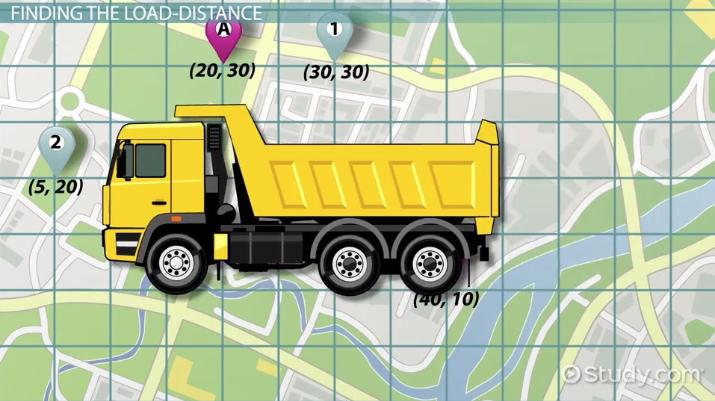Business Courses / Course / Chapter

# Using the Load Distance Technique for Site Planning

Lesson Transcript
Instructor: Kevin Newton

Kevin has edited encyclopedias, taught history, and has an MA in Islamic law/finance. He has since founded his own financial advice firm, Newton Analytical.

The load-distance technique is often used to compare locations for new factories and overall site planning. Find out how to calculate the cost of getting resources from suppliers to find the best site. Updated: 12/13/2021

Let's say that you were trying to analyze potential locations for a new widget factory. You have it down to a short list of two sites. Both are relatively similar in just about every measurable way. You have equal access to a workforce, highways, and customer bases, and the taxes at each location are comparable. What will ultimately be the deciding factor is the distance to your suppliers. In this lesson, we'll make use of the load-distance technique to compare distances to suppliers relative to the number of shipments needed from them to figure out where to best place our new widget factory.An error occurred trying to load this video.

Try refreshing the page, or contact customer support.

Coming up next: Location Factor Rating & Center of Gravity in Site Planning

### You're on a roll. Keep up the good work!

Replay
Your next lesson will play in 10 seconds
• 0:42 Straight Line Distance Formula
• 1:38 Finding the Distance of Sites
• 5:31 Lesson Summary
Save Timeline
Autoplay
Autoplay
Speed Speed

## Straight Line Distance Formula

In order to make use of the load-distance technique, I need to take you back to high school geometry. No, you won't have to use the Pythagorean theorem, but instead the straight line distance formula. If you don't remember, it is the square root of the sum of the difference between the x values of two sites, squared, plus the difference between the y values of two sites, squared. Here's the formula on screen, actually (see video). In order to use the load-distance technique, we'll be using this formula a lot. Also, that means we'll be placing the whole region to be examined on a giant Cartesian plane. It doesn't matter where you start, as long as the relative distances are the same, and you keep everything positive. As a tip, however, I'll advise you to use miles as each unit. It just makes things easier to understand. In other words, don't go shifting the grid after we begin!

## Finding the Distance of Sites

So your widget factory has two potential locations, represented on the graph at points (20, 30) and (40, 10). What? What graph? In order to use the load-distance technique, we have to overlay the map of the area onto a coordinate graph. We'll refer to these as A and B. Meanwhile, you have two major suppliers. It's a little known fact that widgets are produced from gizmos and gadgets, but now you know. The gizmo source is located at (30, 30), while the gadget source is located at (5, 20). We will refer to these as 1 and 2. Also, note that just because the first factory and the gizmo source both have y coordinates of 30 that they are not located at the same place. Now, we get to find the distance between each potential factory site and each supplier location. We'll do the first two together, then for the sake of time, I'll give you the last two.

For the distance from factory A to supplier 1, first subtract the x value of the supplier from that of the factory. That's 20-30, or negative 10. Squared, that's 100. We'd add that to the difference of the y value of each, but since 30 - 30 is zero, we only need to find the square root of 100. That comes out to 10. That's the distance from factory A to supplier 1.

For the distance from factory A to supplier 2, first subtract the x value of the supplier from that of the factory. Once again, that's 20 - 5, or 15, then squared, which gives us 225. We do have to subtract 20 from 30, so 30 - 20 is 10, when squared gives us 100. Add 225 and 100 together, and you get 325. Take the square root, and you end up with 18.03.

To unlock this lesson you must be a Study.com Member.

### Register to view this lesson

Are you a student or a teacher?

Back

### Resources created by teachers for teachers

Over 30,000 video lessons & teaching resources‐all in one place.Video lessonsQuizzes & WorksheetsClassroom IntegrationLesson Plans

I would definitely recommend Study.com to my colleagues. It’s like a teacher waved a magic wand and did the work for me. I feel like it’s a lifeline.

Jennifer B.
TeacherCreate an account to start this course today
Used by over 30 million students worldwide